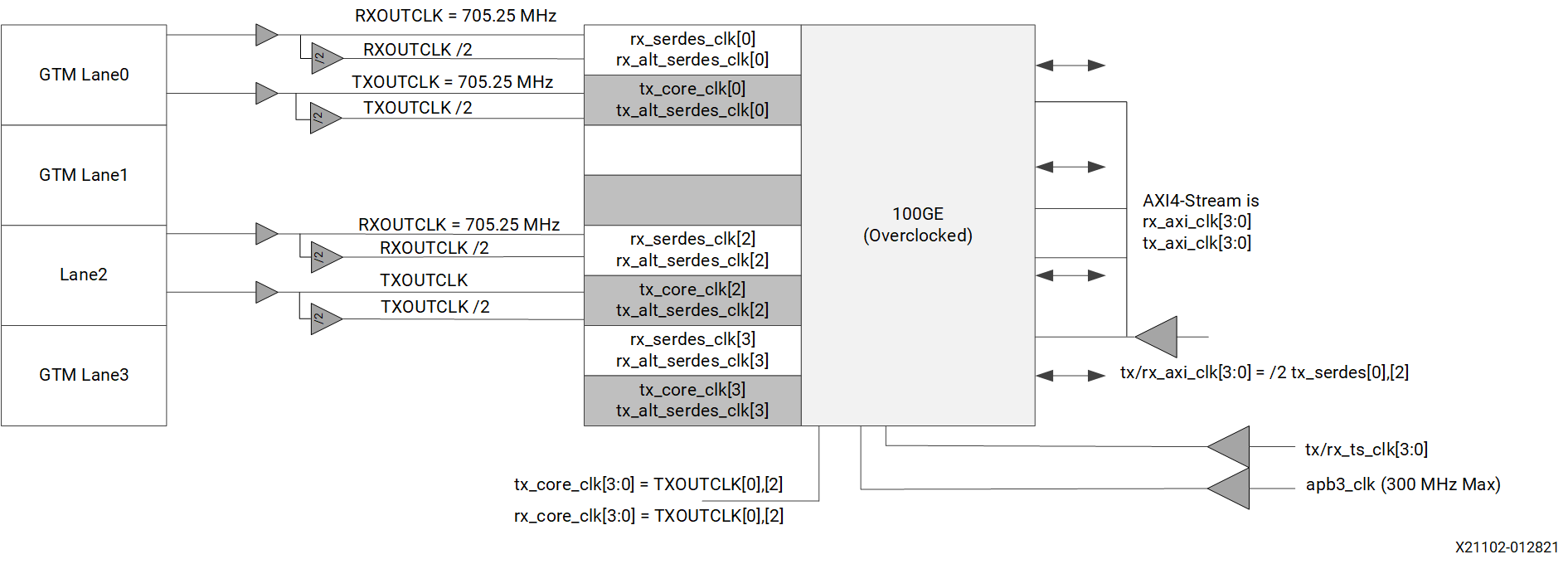# Standard Ethernet MAC + PCS (Low Frequency AXI4-Stream) - 1.3 English

Document ID
PG314
Release Date
2021-02-05
Version
1.3 English

## Example 2a

• 4 × 10/25GE (4 × 10.3125/4 × 25.78 Gb/s)
• Low Latency AXI Operating Mode
Figure 1. Example Diagram 2a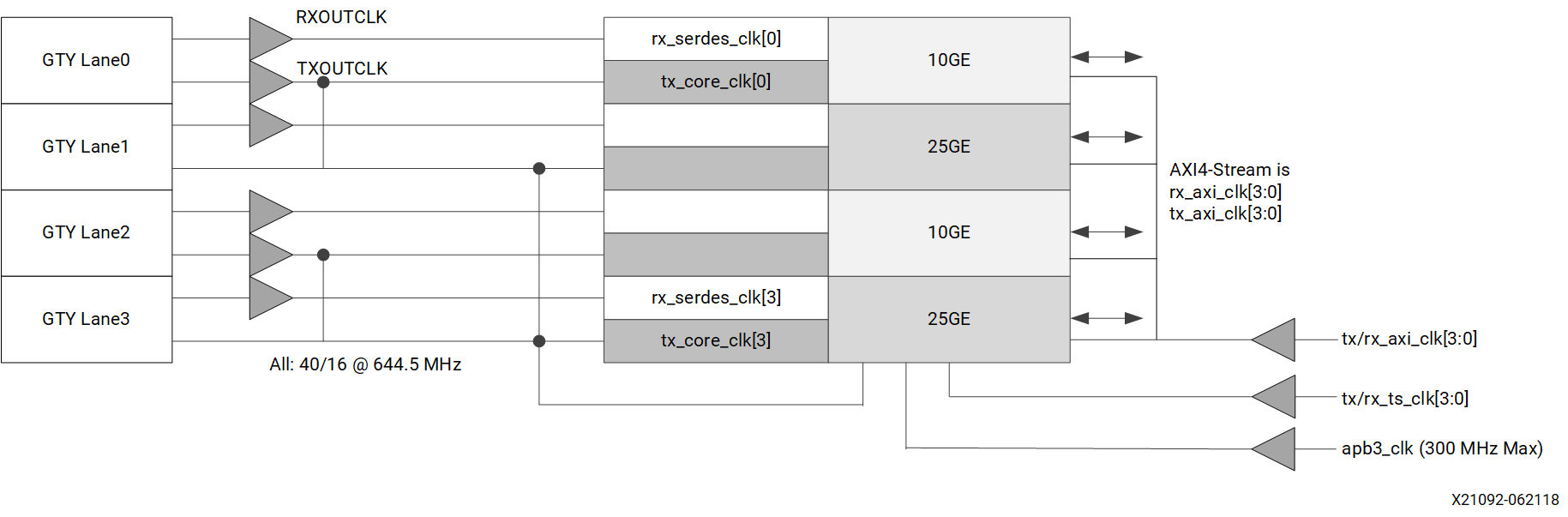## Example 2b

• 1 × 50GE (1 × 53.12 Gb/s)
• 2 × 10/25GE 2 × 10.31/25.78 Gb/s)
• 50GE IEEE 802.3cd
• Low Frequency AXI Operating Mode

In the example diagram 2b, the unused lane can be dark but still connected for switchable use cases.

Figure 2. Example Diagram 2b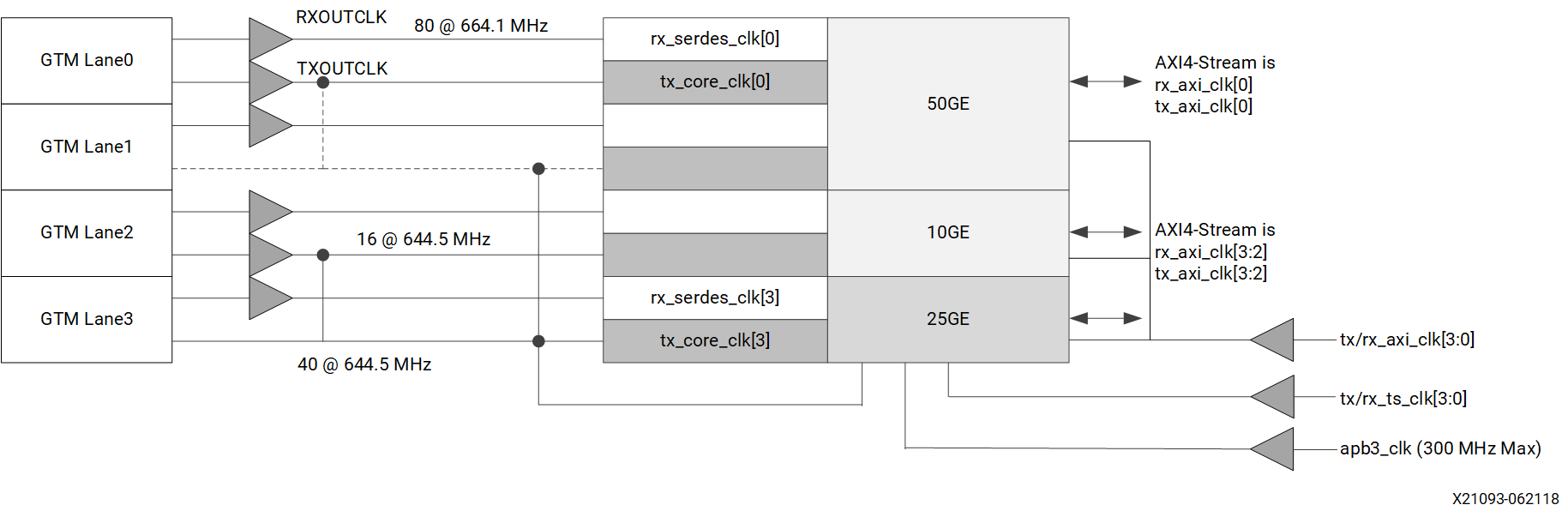## Example 2c

• 1 × 50GE (2 × 26.56 Gb/s)
• 2 × 10/25GE 2 × 10.31/25.78 Gb/s)
• 50GE IEEE 802.3cd External Gearbox
• Low Frequency AXI Operating Mode
The following shows the clocks used in the example diagram 2c use case:
• 4 × RX SerDes clock
• 2 × TX SerDes clock (and `tx/rx_core_clk[3:0]`)
• 1 × System timer clock
• 3 × AXI4-Stream clock (independent AXI)
• 1 × AXI4-Stream clock (shared AXI) 1 × APB3 clock
• 11 clocks (independent AXI)
• 21 clocks for DD MRMAC (share APB3)
• 9 clocks (shared AXI)
• 17 clocks for DD MRMAC
Figure 3. Example Diagram 2c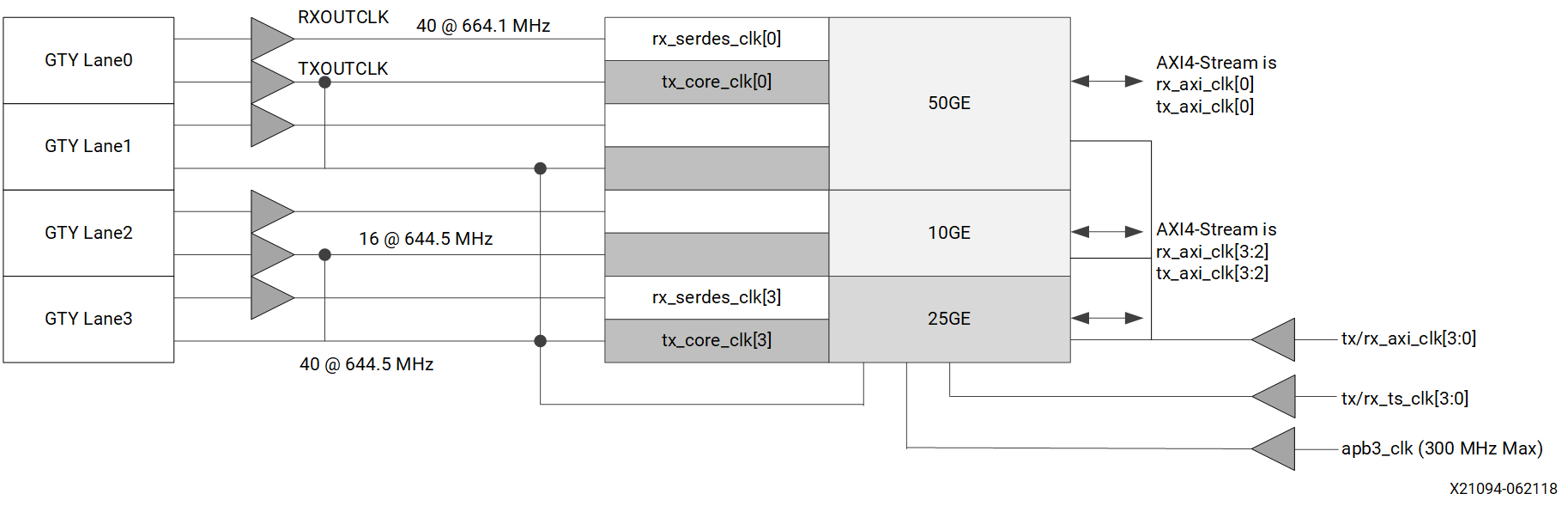## Example 2d

• 1 × 100GE (4 × 25.78/26.56 Gb/s)
• Low Frequency AXI Operating Mode
Figure 4. Example Diagram 2d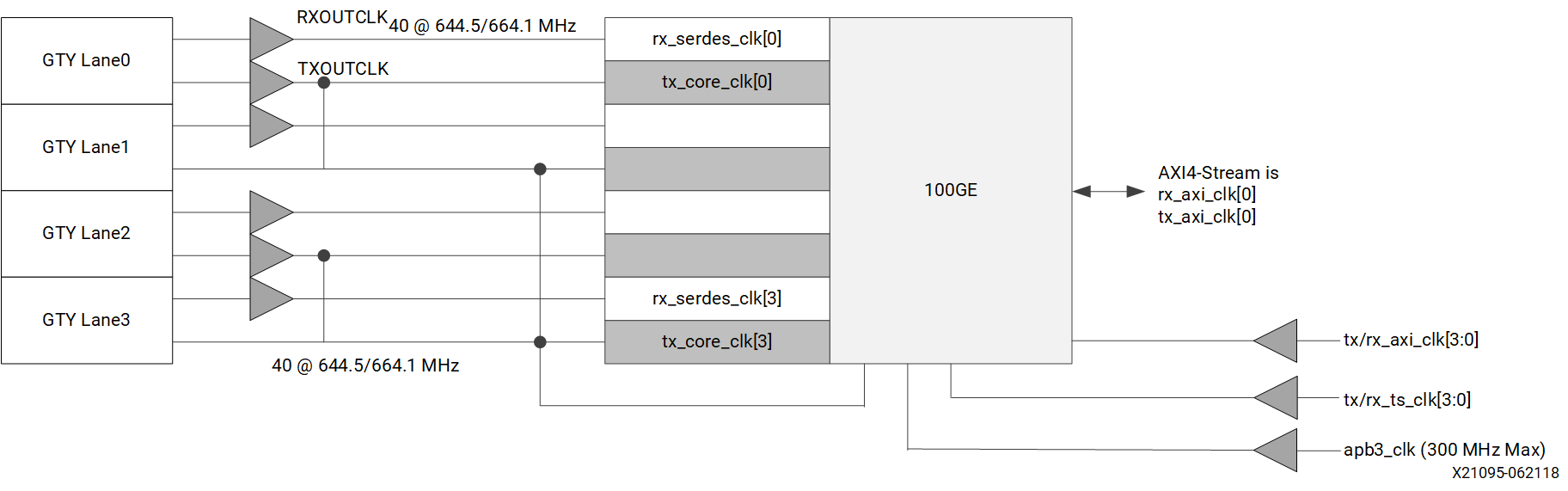## Example 2e

• 4 × 10/25GE (4 × 10.3125/4 × 25.78 Gb/s)
• Low Frequency AXI Operating Mode
• Drive `tx/rx_axi_clk[3:0]` with TXOUTCLK/2
Figure 5. Example Diagram 2e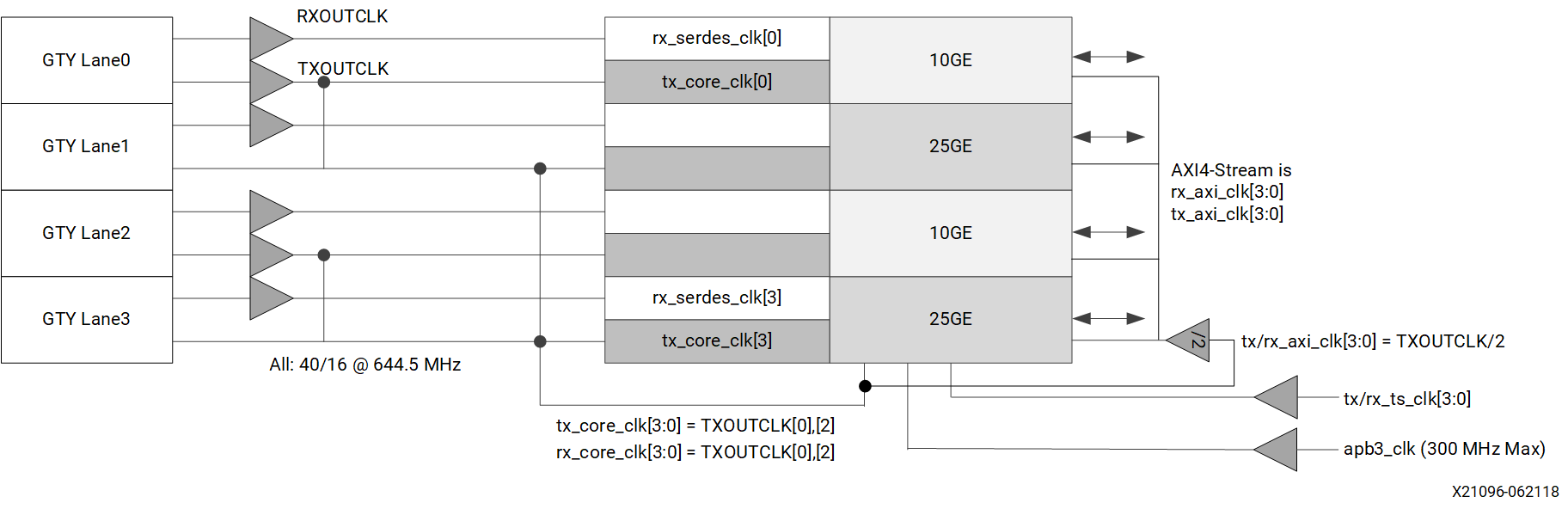## Example 2f

• 1 × 50GE (1 × 53.12 Gb/s)
• 2 × 10/25GE 2 × 10.31/25.78 Gb/s)
• Low Frequency AXI Operating Mode
• 50GE IEEE 802.3cd
Figure 6. Example Diagram 2f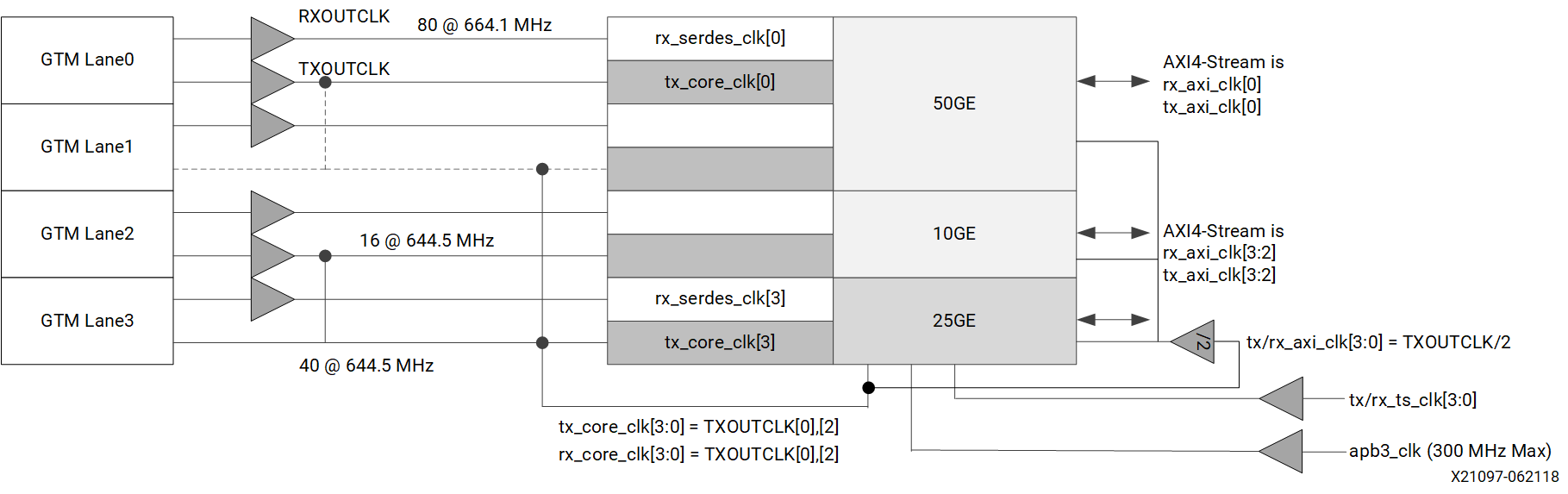## Example 2g

• 4 × 10/25GE (4 × 10.3125/4 × 25.78 Gb/s)
• Independent Clock Lanes
• Low Frequency AXI Operating Mode
• Drive `tx/rx_axi_clk[3:0]` with TXOUTCLK/2
Figure 7. Example Diagram 2g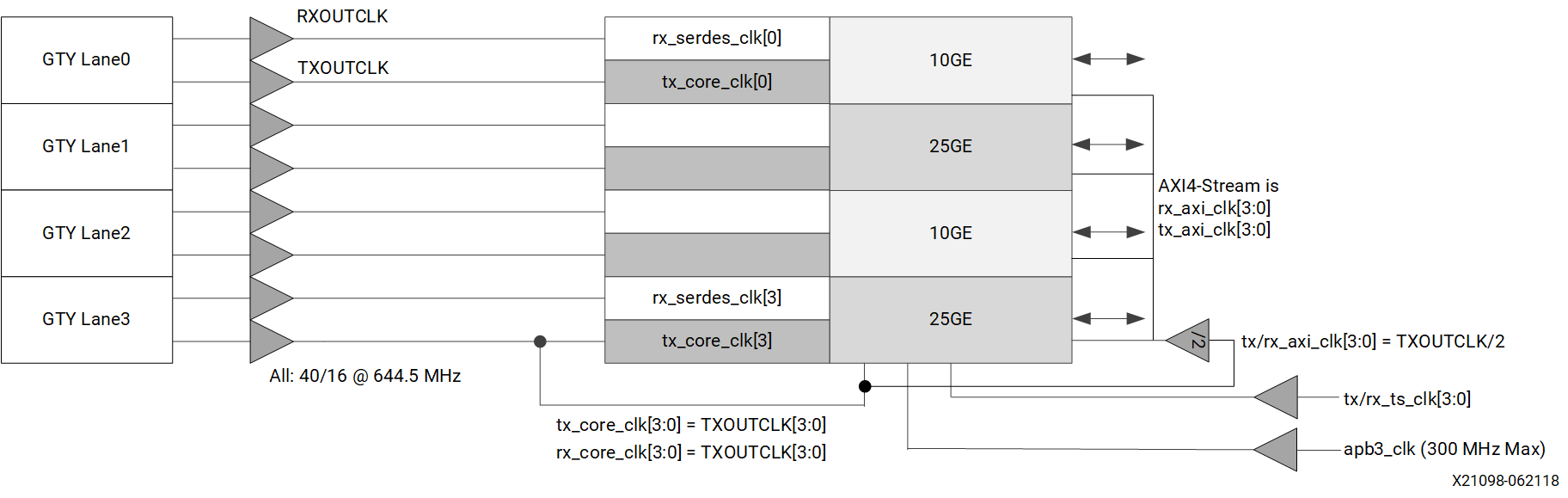## Example 2h

• 4 × 10/25GE (4 × 10.3125/4 × 25.78 Gb/s)
• Independent Clock Lanes
• Low Frequency AXI Operating Mode
Figure 8. Example Diagram 2h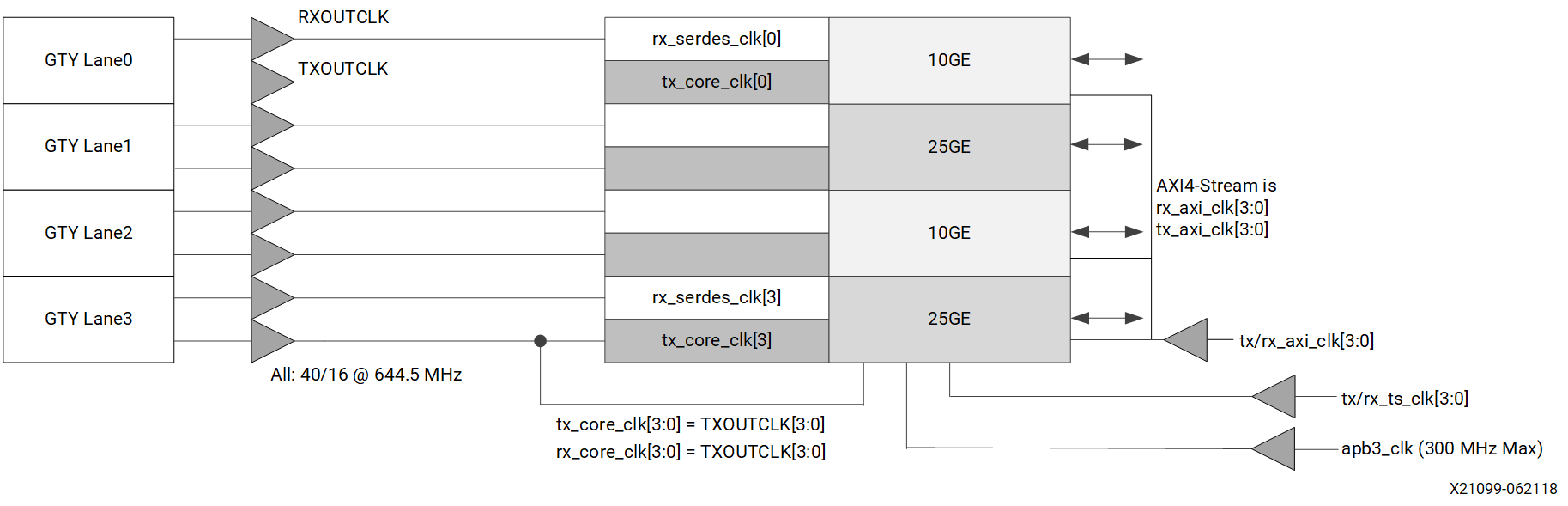## Example 2i

• 4 × 10/25GE (4 × 10.3125/4 × 25.78 Gb/s)
• Low Speed PCS Data (-1LP)
• Low Frequency AXI Operating Mode

In the example diagram 2i, 25GE Data out 80 @ 322.26 MHz and 10GE Data out 32 @ 323.26 MHz. TX/RXOUTCLK is ×2 data rate and local /2 bufg in RCLK region generates the clock to capture the SerDes Data.

Figure 9. Example Diagram 2i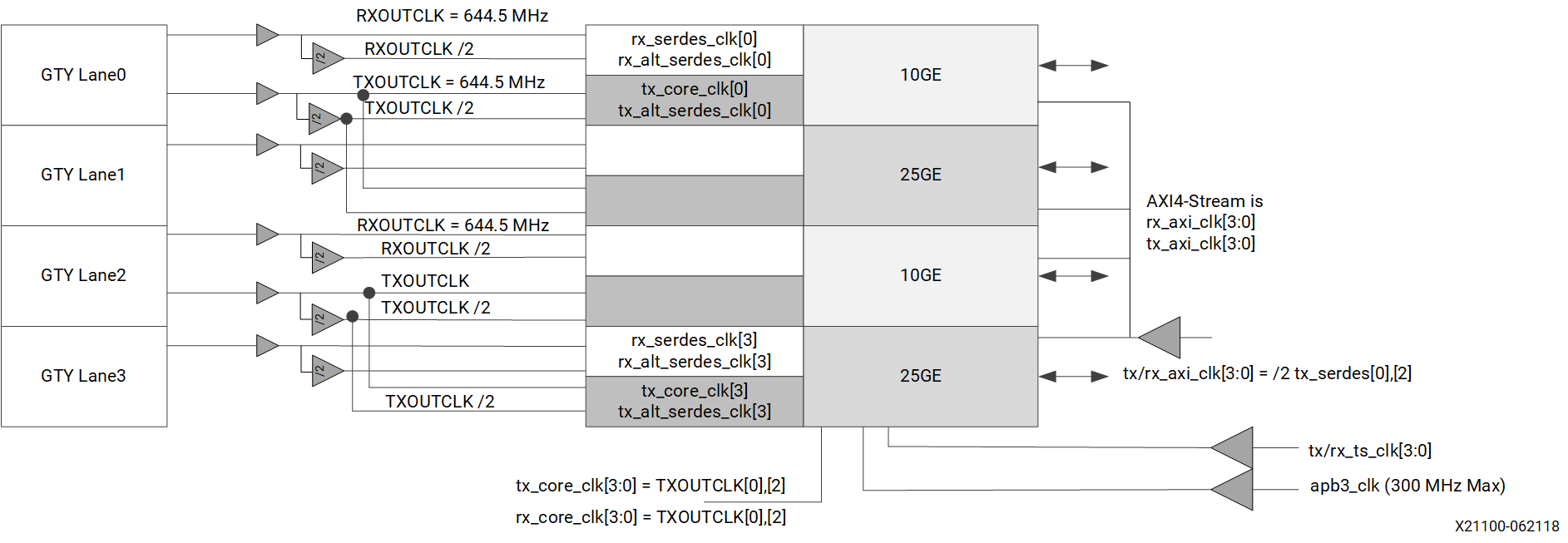## Example 2j

• 1 × 100GE (4 × 28.21 Gb/s) Overclock
• Low Frequency AXI Operating Mode

Each lane has 28.21 Gb/s Data out 80 @ 352.62 MHz. TX/RXOUTCLK is ×2 data rate and local /2 bufg in RCLK region generates the clock to capture the SerDes Data.

Figure 10. Example Diagram 2j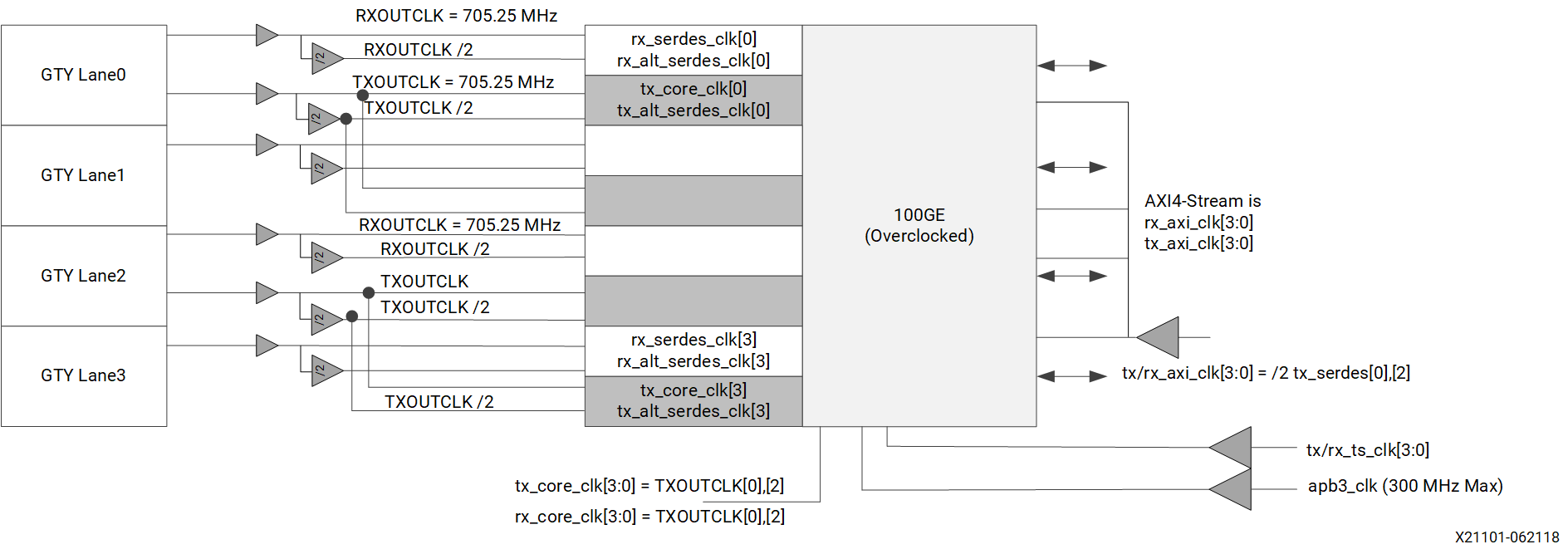## Example 2k

• 1 × 100GE (2 × 56.42 Gb/s) Overclock
• Low Frequency AXI Operating Mode

Each lane has 56.42 Gb/s Data out 160 @ 352.62 MHz. TX/RXOUTCLK is ×2 data rate and local /2 bufg in RCLK region generates the clock to capture the SerDes Data.

Figure 11. Example Diagram 2k